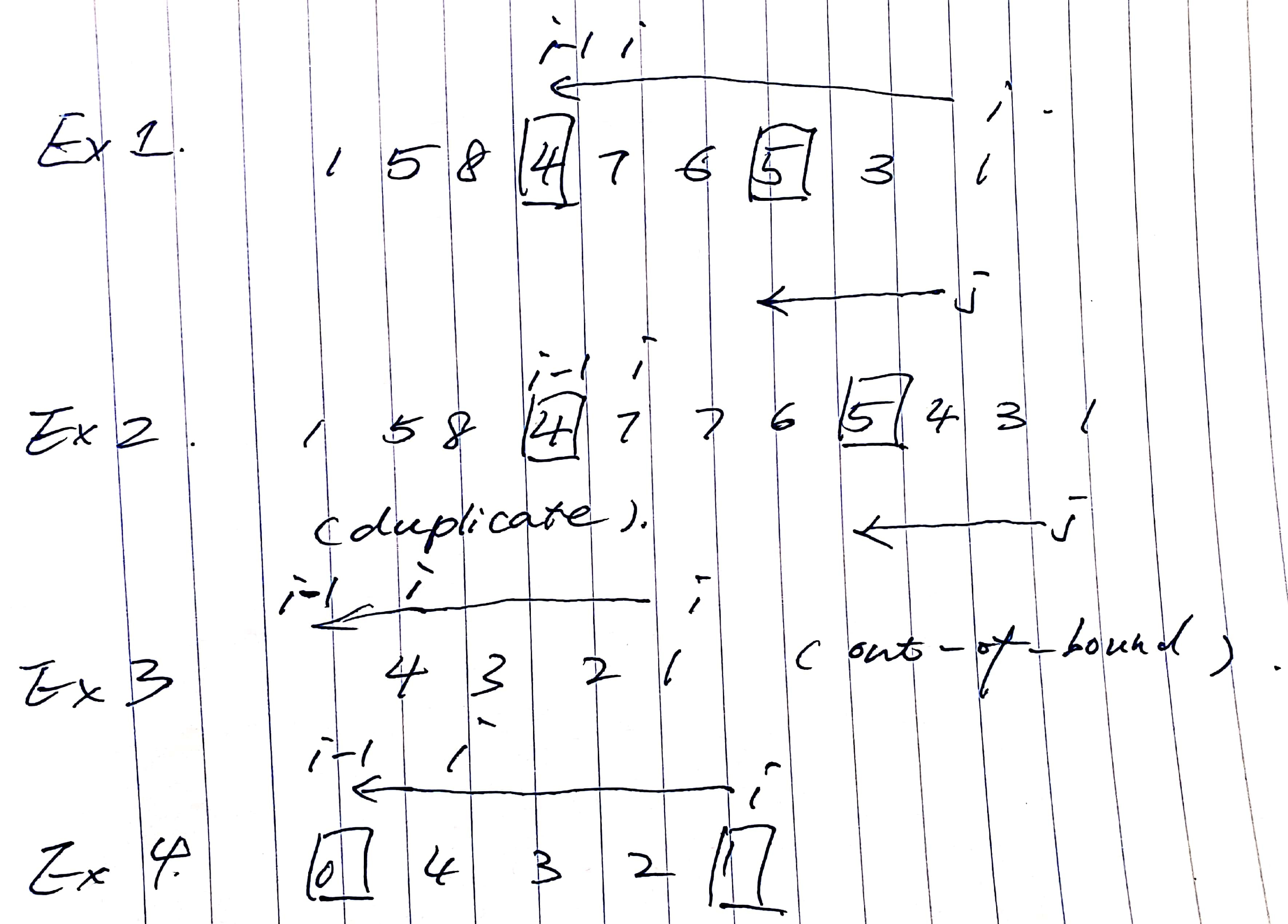# 31. Next Permutation

Reference: LeetCode
Difficulty: Medium

## Problem

Implement next permutation, which rearranges numbers into the lexicographically next greater permutation of numbers.

If such arrangement is not possible, it must rearrange it as the lowest possible order (ie, sorted in ascending order).

The replacement must be in-place and use only constant extra memory.

Here are some examples. Inputs are in the left-hand column and its corresponding outputs are in the right-hand column.

Example:

## Analysis

### Brute-Force

Generate every possible permutation and do comparison.

Time: $O(N!)$
Space: $O(N)$

### Single Pass

Note: This problem is good for index manipulation practice.

Idea: LeetCode Solution

Try these examples:Time: $O(N)$
Space: $O(1)$

CommentJunhao Wang
a software engineering cat